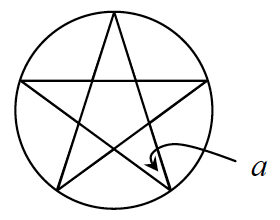### Home > GC > Chapter 12 > Lesson 12.1.5 > Problem12-47

12-47.A regular pentagram is a 5-pointed star that has congruent angles at each of its outer vertices. Use the fact that all regular pentagrams can be inscribed in a circle to find the measure of angle a at right.

Find the measure of each of the intercepted arcs.

$\frac{360^\circ}{5} = 72^\circ$

The degree measure of an inscribed angle is half the measure of the intercepted arc.

$\frac{72^\circ}{2}$

$= 36°$